Structures in C Programming

Introduction

When we write programs to address real world requirements, variables and their datatypes are not limited single type of variables. We will have various requirement to have different types of variables clubbed together and called using a single name. For example students in a class. When we say student,  we have lot of informations related to him needs to be stored and accessed like his ID, name, age, his class etc. Here we can store all these informations in separate variables. This will work fine when there is only one student. Each of his informations can be stored in respective variables with correct datatypes.

int intStdId;
char chrStdName;
int intAge;
char chrClassId;

But in a class or college, number of students is not limited to one. There will be lot many students. As we have seen already, when we have multiple data to be stored of same kind, we usually use arrays. Hence we might have to create arrays for each of the variables above. It can  now store the data for any number of  students in class / college.

int intStdId ;
char chrStdName;
int intAge;
char chrClassId;

In above illustration, we are storing 100 student informations. What if we get more number of students latter? This array will not be sufficient. We have to modify all the array variables that are used to store student information to store more number of students. In addition, we have to modify the code everywhere we use these arrays, for loops(to increase the index), conditions etc.

Apart from above issue, there is one more issue of mapping of student details. All these array variables are independent of each other, even though they are used to store student details. We know that they are used for student details, since we are coding it. Also, we might have followed the rule that first element of all the array variable contains the detail of one student, next subsequent elements store the details about next subsequent students. But what is the guarantee that they are always stored in the same way? In these types of non-related arrays, there is always high possibility of mixing the data among its elements. Hence there will be always fear of having wrong student details.

Constants in C Programming

All these problems make us to think of using some other way of storing and accessing the data, without missing data or creating any wrong data. Here we would always prefer to have individual student details stored in one variable rather than multiple variables. If it is stored in one variable, we will not have any cross mapping of student details. But when we say one variable, storing all ids, names, age, class etc in a variable like a long string will not help. Extracting each value from the string would be a challenge. Hence we need to have variables in such a way that,

• Each element related to the student should be identified clearly
• There should not be any cross mapping or wrong interpretation of data.
• Each element of student should have different variables with their respective datatype, but still we should be able to identify by them by using single name.

All these are possible by using a special datatype in C called structures. These are the derived datatypes in C, which contains variables the primitive datatypes and derived datatypes under one name, which is another variable.

In our example above, we have student id, student name, age and class ids are all related to the student and we would like to call of them as student. Hence we create a structure student, which will have its elements as id, name, age and class with primitive/ non-primitive datatypes.

If we have multiple students, then we can create array of structure student, which makes any copies of students. Hence there is no fear of cross mapping of data. Each element of structure array will contain its own elements, which cannot be mixed with other element of structure array.

Below diagram about the structure will clear these concepts.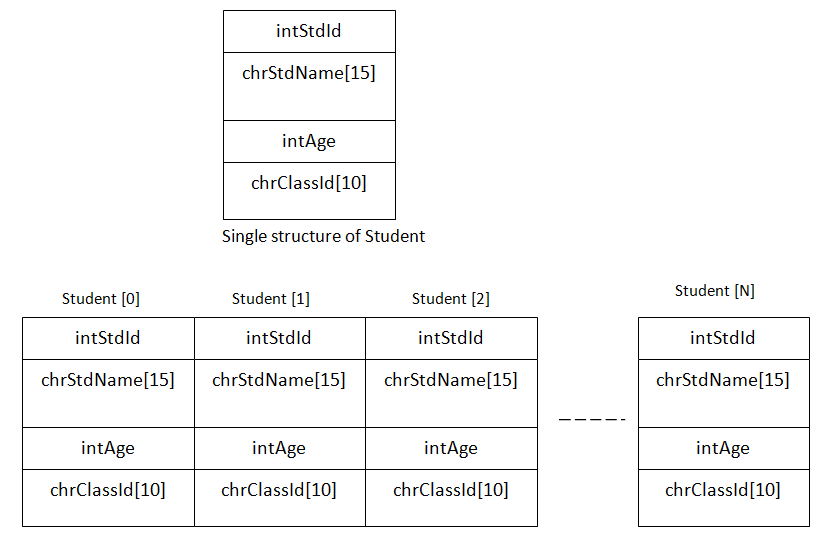Thus, when we say student, we will have all the information for the student stored at location 0 and so on. We can even have another structure within one structure.

These excellent concepts of structure make C more powerful to address all real life scenarios.

There are many situations where we use structures: address, employee details, author details, book informations etc. Let us now see how to create structures.

Declaration and Type

As we discussed above, any structure will have one or more primitive or non-primitive datatype within it. Hence it will be of the form of wrapper.

The general syntax for creating structure is given below :

struct struct_name{
datatype variable1;
datatype variable2;
….
datatype variableN;
};

Here struct keyword is used to indicate that it is a structure type of variable, followed by structure name. Inside it will have its element variables with their respective datatypes.

Register Storage Class in C Programming

Student structure above can be declared as below :

struct student{
int intStdId;
char chrStdName;
int intAge;
char chrClassId;
};

Here student structure has 4 elements – id, name, age and class id, with its own data type.

Declaring a structure creates a skeleton of the structure. It does not represent the real variable. It creates only the skeleton for the creating variable. This student structure above will not act as a variable. But it creates a provision to declare any other variable as student. It is similar to creating a user defined datatype and the using that datatype to declare the variables.

That is if we need to assign any student details to above structure, first we need to create a structure variable whose datatype is student, i.e.;

struct student struct_std1, struct_std2;

Now struct_std1 and struct_std2 are called instance of the structure student. Suppose we need to create an array of students with same structure. This can be done in the same way as we declare an array. Here we use structure type instead of any primitive datatypes.

struct student struct_StdArr; // creates an array of structure with 10 elements

Above one is a simple structure. We can have complex structure – structure within a structure. This is called nested structures. Consider the same student structure with same elements along with their address. Here Address cannot be a single variable. It will have door#, street#, city, state and pin code. Hence it can be considered as another structure. Since this is related to student, address structure needs to be declared within student structure.

struct student{
int intStdId;
char chrStdName;
int intAge;
char chrClassId;
int intDoorNum;
char chrstreet;
char chrCity;
char chrState;
int intPincode;
};
};

Now instance of the student structure can be created in the same way as we created earlier. This new student structure defines a new definition for the student structure type with address structure within it,  i.e.;

struct student struct_std1, struct_std2;

We can even create an instance of the structure while declaring them itself.

struct student{
int intStdId;
char chrStdName;
int intAge;
char chrClassId;
}struct_std1, struct_std2; // 2 instances are created

struct student{
int intStdId;
char chrStdName;
int intAge;
char chrClassId;
int intDoorNum;
char chrstreet;
char chrCity;
char chrState;
int intPincode;
}struct_std; //instance of student

We can even create address structure separately outside the student structure and then can have instance of address created within student. This also works in the same way as above.
int intDoorNum;
char chrstreet;
char chrCity;
char chrState;
int intPincode;
};
struct student{
int intStdId;
char chrStdName;
int intAge;
char chrClassId;
}struct_std1; // one method of creating instance
struct student struct_std2; //another method of creating instance

Accessing Elements of Structure

We have declared a structure and created its variables / instances. Now the question is how to access the elements within the structure. Since it is wrapped under single name and it should be uniquely accessed for each student, accessing its element should also be uniquely. We cannot directly access the elements like we access any other variables.

C Inputs and Outputs in C Programming

The requirement of the structure makes it to append the structure variable name before its element variables. This makes it to uniquely access its elements and stops it from any cross mapping.

In our example of student structure, we can access its elements like below :

struct student struct_std1, struct_std2;

struct_std1.intStdId = 100;
strcpy(struct_std1.chrStdName ,“Rose”);
struct_std1.intAge = 20;
strcpy(struct_std1.chrClassId ,“CLS_001”);

struct_std2.intStdId = 200;
strcpy(struct_std1.chrStdName,“Mathew”);
struct_std2.intAge = 22;
strcpy(struct_std2.chrClassId , “CLS_232”);

Here we have two structure variables struct_std1 and struct_std2. Their respective elements are assigned values by accessing them by appending their respective structure variable names – struct_std1.intStdId, struct_std1.chrStdName, struct_std1.intAge, struct_std1.chrClassId etc.

Values can also be assigned directly like we do with any other normal variables. Here string values are assigned using strcpy function, since structure element is a string variable and does not allow us to directly assign the value. More above strcpy will be discussed in latter topics.

If we need to print its values, then we can use it in the same way.

#include <stdio.h>
#include <string.h>

void main() {
struct student{ // if this structure needs to be accessed by other functions too, then place this structure definition outside the main function
int intStdId;
char chrStdName;
int intAge;
char chrClassId;
int intDoorNum;
char chrstreet;
char chrCity;
char chrState;
int intPincode;
};
};
struct student struct_std1, struct_std2;

struct_std1.intStdId = 100;
strcpy(struct_std1.chrStdName, "Rose");
struct_std1.intAge = 20;
strcpy(struct_std1.chrClassId, "CLS_001");
struct_std1.intDoorNum = 121;
strcpy(struct_std1.chrState, "Bangalore");

struct_std1.intStdId = 200;
strcpy(struct_std1.chrStdName, "Mathew");
struct_std1.intAge = 22;
strcpy(struct_std1.chrClassId, "CLS_232");

printf("\nValue of student Id in struct_std1 is : %d", struct_std1.intStdId);
printf("\nValue of student Name in struct_std1 is : %s", struct_std1.chrStdName);
printf("\nValue of student age in struct_std1 is : %d", struct_std1.intAge);
printf("\nValue of student class  in struct_std1 is : %s", struct_std1.chrClassId);
printf("\nValue of Door Number in struct_std1 is : %d", struct_std1.intDoorNum);

printf("\nValue of student Id in struct_std2 is : %d", struct_std2.intStdId);
printf("\nValue of student Id in struct_std2 is : %s", struct_std2.chrStdName);
printf("\nValue of student Id in struct_std2 is : %d", struct_std2.intAge);
printf("\nValue of student Id in struct_std2 is : %s", struct_std2.chrClassId);
}

Below is the output of above program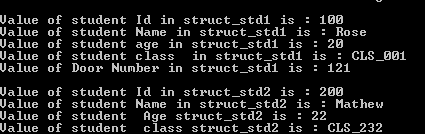Here we can notice that the structure element of address is accessed as if they are direct elements of student. This is because, address structure is within the student structure.

This is one of the examples of accessing the structure within a structure. There are several ways to create structures and accessing them.

Storage Classes in C Programming

Some other methods of initializing and accessing the structure elements are shown below :

struct student{
int intStdId;
char chrStdName;
int intAge;
char chrClassId;
}struct_std1 = { 100, "Rose", 20, "CLS_001" }; // creating an instance and initializing its elements
printf("\nValue of student Id in struct_std1 is : %d", struct_std1.intStdId);
printf("\nValue of student Name in struct_std1 is : %s", struct_std1.chrStdName);
printf("\nValue of student age in struct_std1 is : %d", struct_std1.intAge);
…

struct student{
int intStdId;
char chrStdName;
int intAge;
char chrClassId;
}struct_std1 // creating an instance
struct student struct_std1 = { 100, "Rose", 20, "CLS_001" }; // initializing its elements
printf("\nValue of student Id in struct_std1 is : %d", struct_std1.intStdId);
printf("\nValue of student Name in struct_std1 is : %s", struct_std1.chrStdName);
printf("\nValue of student age in struct_std1 is : %d", struct_std1.intAge);
…

struct student{
int intStdId;
char chrStdName;
int intAge;
char chrClassId;
int intDoorNum;
char chrstreet;
char chrCity;
char chrState;
int intPincode;
}struct_std = { 100, "Rose", 20, "CLS_001", { 121, "MS Road", "Bangalore", "KA", 560034 } };
printf("\nValue of student Id in struct_std is : %d", struct_std.intStdId);
printf("\nValue of student Name in struct_std is : %s", struct_std.chrStdName);
printf("\nValue of Door Num in struct_std is : %d", struct_std. struct_addr. intDoorNum);
printf("\nValue of State in struct_std is : %s", struct_std. struct_addr. chrState);

In this example, address instance is appended because we have created its instance. Since instance of inner structure is created, we cannot directly access their elements. We need to access inner structure elements via instance of inner structure like above example – struct_std. struct_addr. intDoorNum.
int intDoorNum;
char chrstreet;
char chrCity;
char chrState;
int intPincode;
};
struct student{
int intStdId;
char chrStdName;
int intAge;
char chrClassId;
}struct_std1; // one method of creating instance
struct student struct_std2; //another method of creating instance
printf("\nValue of student Id in struct_std1 is : %d", struct_std1.intStdId);
printf("\nValue of student Name in struct_std1 is : %s", struct_std1.chrStdName);
printf("\nValue of student age in struct_std1 is : %d", struct_std1.intAge);
printf("\nValue of Door Num in struct_std is : %d", struct_std1. struct_addr. intDoorNum);

printf("\nValue of student Id in struct_std2 is : %d", struct_std2.intStdId);
printf("\nValue of student Name in struct_std2is : %s", struct_std2.chrStdName);
printf("\nValue of student age in struct_std2 is : %d", struct_std2.intAge);
printf("\nValue of Door Num in struct_std is : %d", struct_std2. struct_addr. intDoorNum);

Here both the instances of student access inner structure instance with the same name. they do not overlap here as struct_addr though structure instance, it represents a variable / element of student structure.

Above are all different methods of creating structure instances, initializing them and accessing them.  Suppose we need to create array of structures. Then we create instance as array in any of the method above.

#include <stdio.h>
#include <string.h>

void main() {

struct student{
int intStdId;
char chrStdName;
int intAge;
char chrClassId;
int intDoorNum;
char chrstreet;
char chrCity;
char chrState;
int intPincode;
};

struct student struct_std;

//initializing different elements of array of structures
struct_std.intStdId = 100;
strcpy(struct_std.chrStdName, "Rose");

printf("\nstruct_std values");
printf("\nValue of student Id in struct_std is : %d", struct_std.intStdId);
printf("\nValue of student Name in struct_std is : %s", struct_std.chrStdName);
printf("\n\nstruct_std values");
printf("\nValue of Door Num in struct_std is : %d", struct_std.struct_addr.intDoorNum);
printf("\n\nstruct_std values");
printf("\nValue of State in struct_std is : %s", struct_std.struct_addr.chrState);
}

We can have pointers to the structure instance. We are creating structure variable as pointers, then we should access the structure members using ‘→’ instead of ‘.’.
#include <stdio.h>
#include <string.h>

void main() {

struct student{
int intStdId;
char chrStdName;
int intAge;
char chrClassId;
};
struct student std;// creating normal structure variable
struct student *struct_std1; // creating a structure pointer

// Initializing the structure elements
std.intStdId = 100;
strcpy(std.chrStdName, "Rose");
std.intAge = 20;
strcpy(std.chrClassId, "CLS_001");

struct_std1 = &std; // Make structure pointer to point to structure std

//Access the structure pointer elements
printf("\nValue of student Id in struct_std is : %d", struct_std1->intStdId);
printf("\nValue of student Name in struct_std is : %s", struct_std1->chrStdName);
printf("\nValue of student age in struct_std is : %d", struct_std1->intAge);
printf("\nValue of student Class in struct_std is : %s", struct_std1->chrClassId);
}

Below is the Output of Above Program

Data Types in C programming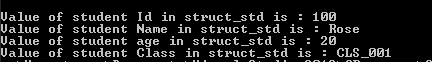Arrays of Structures

We have discussed so far how to handle the requirement to club different types of elements into one name using structure. This structure defined so far is able to handle the data for single student. The structure student, though defined to have id, name, age and class, it holds the data about single student. When we add another student to same structure variable, older information is overwritten. Hence we have to declare another structure variable of type student to handle another student. But what will happen when number of students is more, say 50 or 100 or even more? It is difficult to create so many structure variables and handle them in a code. It reduces the readability of the code as well as increases the complexity in the code. Hence C combines the feature of structure with the features of array. In other words C allows us to create an array of structures. It is created in the same way as we create an array of integers or characters.

We declare an array of type structure to create an array of structures like below :

struct student{
int intStdId;
char chrStdName;
int intAge;
char chrClassId;
};
struct student std;// creating an array of structures with 10 structure elements

Above set of code creates an array of 10 elements. But the elements of the array are structures of type student. That means each element of the array will have 33 bytes of memory allocated to hold id, name, age and class of each students.

Below diagram shows an array of structures with 3 elements. Though individual elements of the structure are represented below, different colored blocks form single elements of the array. Since each element is a structure, memory allocated for each element is divided among the elements of the structure. Each array elements are placed one after the other in memory.

Automatic Storage Class in C programming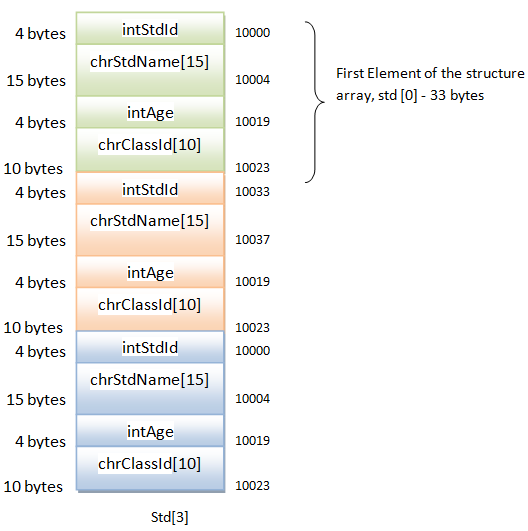We can access array of structures using the ‘.’ Operators like we access any structure elements. Since it is an array, we have to specify array index to represent which element of the array that we are accessing.

Std.intStdId = 100; // initializes the intStdId member of first element of array of structure
Std.StdName =”Mike”;// initializes the StdName member of third element of array of structure

Memory Allocation in Structure

Like any other variable, structure also needs memory to be allocated for it to store the data. But memory allocated for any structure cannot be constant like any other datatypes like int, float, long, char etc. This is because; structure can have one or more elements which are of different types. Hence its size and memory varies according to the elements in the structure.

In structure, individual elements are allocated memory according to their datatype and the memory allocated for the structure will be the sum of the memory allocated for the individual elements of the structure. For example, consider a simple student structure.

struct student{
int intStdId;
char chrStdName;
int intAge;
char chrClassId;
}struct_std;

Here the size of structure variable struct_std is given by the sum of size of individual elements – intStdId, chrStdName, intAge and chrClassId. i.e.;

Size of (intStdId)+ sizeof (chrStdName)+ size of( intAge) + size of(chrClassId)
= 4 + 15+ 4+ 10
=33bytes.

From this we can understand that even though individual members occupy their individual spaces in the memory, when we declare a structure variable, struct_std, we need 33bytes of continuous memory. It is then divided to accommodate its members.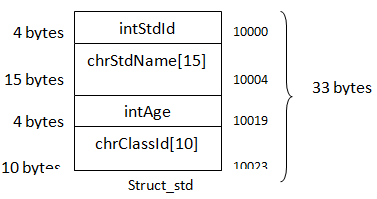Consider a nested structure to calculate its memory allocation.

struct student{
int intStdId;
char chrStdName;
int intAge;
char chrClassId;
int intDoorNum;
char chrstreet;
char chrCity;
char chrState;
int intPincode;